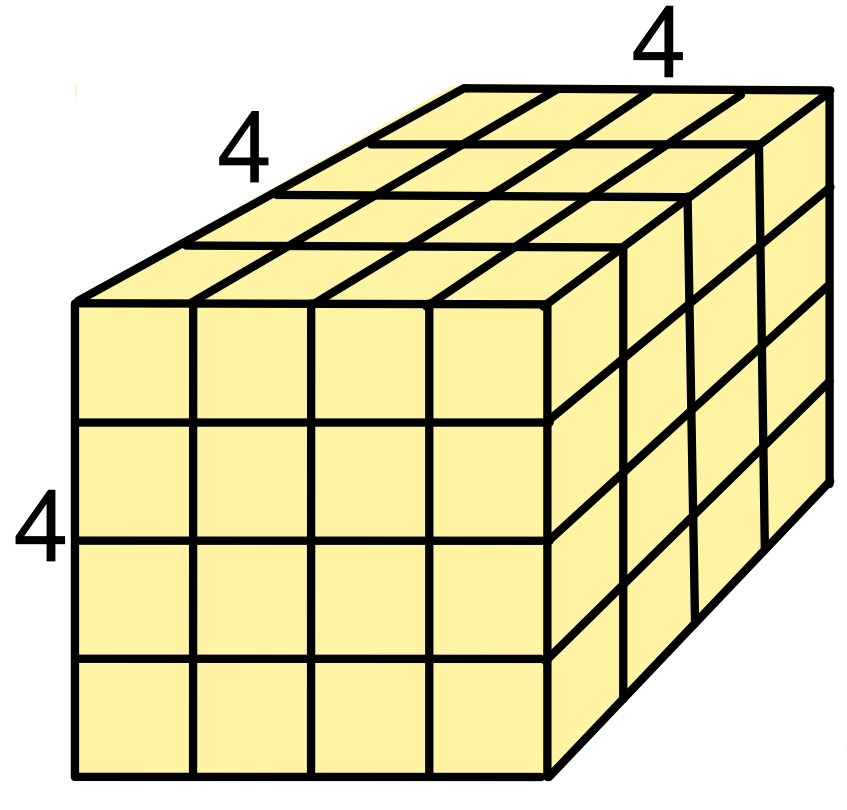Cubes

## Cubes

Multiplying a number by itself, then multiplying by itself again gives the cube of a number:

4 x 4 x 4 = 43 = 64.

The small 3 (the index) after the number indicates that it is being cubed.A negative number that is cubed will result in a negative number:

-33 = -3 x -3 x -3 = -27

A number less than 1 will result in a smaller number:

0.23 = 0.2 x 0.2 x 0.2 = 0.008

Fractions can also be cubed:

(frac(1)(2))3 = frac(1)(2) x frac(1)(2) x frac(1)(2) = frac(1)(8)

## Example 1

What is 0.53?

Work out 5 x 5 x 5 = 125: there were 3 x 1 decimal places in the original numbers, make sure that there are three decimal places in the answer = 0.125.